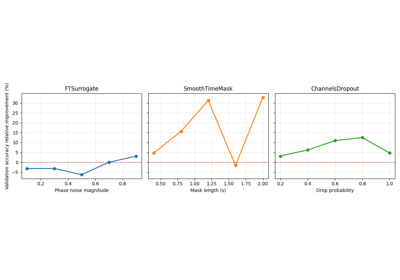# braindecode.augmentation.FTSurrogate#

class braindecode.augmentation.FTSurrogate(probability, phase_noise_magnitude=1, channel_indep=False, random_state=None)[source]#

FT surrogate augmentation of a single EEG channel, as proposed in .

Parameters
• probability (float) – Float setting the probability of applying the operation.

• phase_noise_magnitude (float | torch.Tensor, optional) – Float between 0 and 1 setting the range over which the phase pertubation is uniformly sampled: `[0, phase_noise_magnitude * 2 * pi]`. Defaults to 1.

• channel_indep (bool, optional) – Whether to sample phase perturbations independently for each channel or not. It is advised to set it to False when spatial information is important for the task, like in BCI. Default False.

• random_state (int | numpy.random.Generator, optional) – Seed to be used to instantiate numpy random number generator instance. Used to decide whether or not to transform given the probability argument. Defaults to None.

References

1

Schwabedal, J. T., Snyder, J. C., Cakmak, A., Nemati, S., & Clifford, G. D. (2018). Addressing Class Imbalance in Classification Problems of Noisy Signals by using Fourier Transform Surrogates. arXiv preprint arXiv:1806.08675.

Methods

get_augmentation_params(*batch)[source]#

Return transform parameters.

Parameters
• X (tensor.Tensor) – The data.

• y (tensor.Tensor) – The labels.

Returns

params – Contains:

• phase_noise_magnitudefloat

The magnitude of the transformation.

• random_statenumpy.random.Generator

The generator to use.

Return type

dict

static operation(X, y, phase_noise_magnitude, channel_indep, random_state=None)#

FT surrogate augmentation of a single EEG channel, as proposed in .

Function copied from cliffordlab/sleep-convolutions-tf and modified.

Parameters
• X (torch.Tensor) – EEG input example or batch.

• y (torch.Tensor) – EEG labels for the example or batch.

• phase_noise_magnitude (float) – Float between 0 and 1 setting the range over which the phase pertubation is uniformly sampled: [0, phase_noise_magnitude * 2 * pi].

• channel_indep (bool) – Whether to sample phase perturbations independently for each channel or not. It is advised to set it to False when spatial information is important for the task, like in BCI.

• random_state (int | numpy.random.Generator, optional) – Used to draw the phase perturbation. Defaults to None.

Returns

• torch.Tensor – Transformed inputs.

• torch.Tensor – Transformed labels.

References

1

Schwabedal, J. T., Snyder, J. C., Cakmak, A., Nemati, S., & Clifford, G. D. (2018). Addressing Class Imbalance in Classification Problems of Noisy Signals by using Fourier Transform Surrogates. arXiv preprint arXiv:1806.08675.

## Examples using `braindecode.augmentation.FTSurrogate`#Searching the best data augmentation on BCIC IV 2a Dataset

Searching the best data augmentation on BCIC IV 2a Dataset